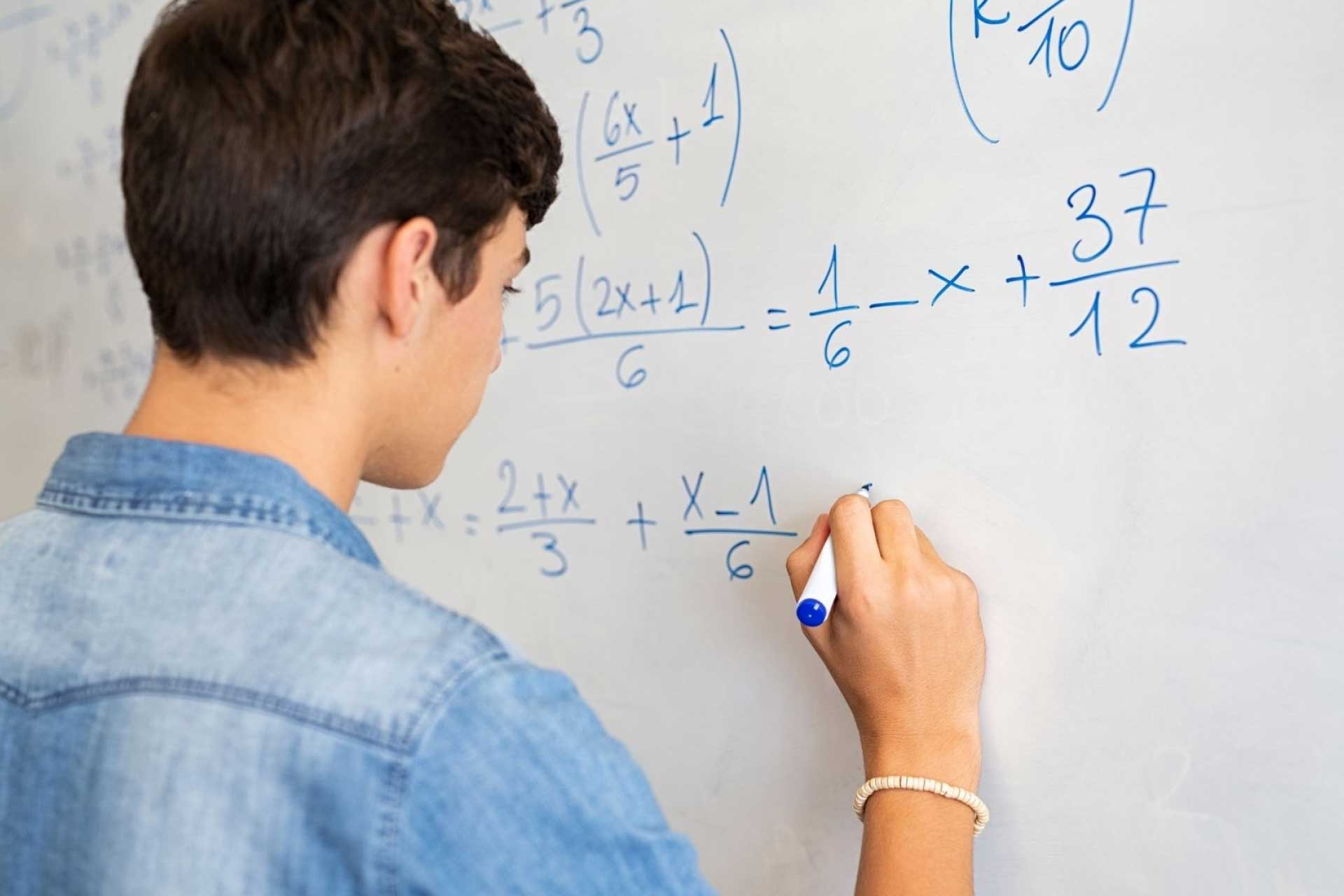# In Mathematics, What Are Parameters?

Parameters are a special type of mathematical variable. A parametric equation contains one or more parametric variables that have multiple possible values. Math tutoring can help students grasp complex concepts in parameters, functions, and values. The value of each parameter is held constant when the function is used. In the statistical branches of mathematics, a parameter is an estimated numerical value for a population characteristic.

## Examples of Parametric Equations

The quadratic equation is a familiar example that can be written as a parametric equation. In the form ax2 + bx + c = 0, a, b, and c are parameters. If the parametric variables are assigned values (such as a = 1, b = 2, c = 3), the equation is no longer parametric. x2 + 2x + 3 is one distinct member of the family of quadratic functions.

Another familiar example is the equation for a straight line drawn on a Cartesian coordinate system. The most general form of the equation is y = mx + b. The variables m and b are usually called the slope and the intercept, respectively. By varying m and b, an infinite number of distinct straight lines can be produced. However, the equation can never produce a parabola or a circle, no matter what combination of m and b is used. The equation is said to produce a family of functions because each function produces the same result – a straight line.

## How Do You Write Parametric Equations?

A parameter may also be used to describe a system of equations. For example, if a ball is thrown and its trajectory is plotted on a Cartesian coordinate system, both the x and y components of the trajectory depend on the time after the ball was thrown and the ball's initial velocity. The equations may look something like x = vt and y = vt - 5t2. In this case, the parameters are velocity and time.

A more advanced application of parameters is the variation of parameters method, which is used to solve differential equations. In this method, the parameters are actually functions that replace unknown constants in the solution to a differential equation. By solving for these parametric functions, the unknown constants can be determined and the general and particular solutions for a differential equation can be found.

## ‍Parameters in Statistics

There are many ways in which parameters are utilized in real-life scenarios, such as in the field of statistics. In statistics, a parameter is an estimate of a given population. Common statistical parameters include mean and standard deviation. These estimates are used in the equations to calculate the test statistic for various statistical tests.

## When Are Parameters Taught in High School?

Students need to have a firm grasp of parameters for success in precalculus and calculus, both of which are widely taught in high schools. Parameters are often taught alongside other mathematical functions in precalculus. Because parameters are taught in precalculus, students will likely learn about them during the second half of their high school careers.

High school students normally take Algebra 1 in ninth grade, geometry in tenth grade, Algebra 2 or trigonometry in eleventh grade, and precalculus or calculus in twelfth grade. However, honors or AP students are likely to take Algebra 1 in eighth grade, geometry in ninth grade, Algebra 2 or trigonometry in tenth grade, precalculus in eleventh grade, and calculus in twelfth grade. This can differ from state to state, as there is some variation in state guidelines regarding the math curriculum taught in high schools.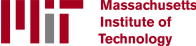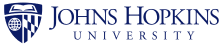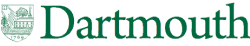## What Are Parametric Equations Used for in Real Life?

Parametric equations are often used to describe types of curves that can be represented on a plane, but these equations are also used when curves on a Cartesian plane cannot be described by a function. Parameters allow you to graph the complete position of an object over time.

In everyday life, parametric equations are useful for measuring circular motion and projectile motion. For example, you can use parameters to measure the curve of a thrown ball or your position on a moving Ferris wheel.

## Are Parametric Equations on the SAT?

The SAT Math section covers a fairly wide range of mathematical topics. Students need to grasp concepts such as operations, algebra, functions, geometry, measurement, data analysis, statistics, and probability in order to succeed on the SAT. The Math section of the SAT includes 19 algebra questions, 17 questions about problem-solving and data analysis, 16 questions related to advanced math (e.g., solving quadratic equations and manipulating polynomials), and 6 questions related to additional math topics (e.g., trigonometry, calculating area and volume, and using theorems related to circles and lines).

The SAT Math questions will likely test students on their ability to create and solve linear equations, analyze quantitative data, apply probabilities in context, create equivalent algebraic expressions, solve problems related to area and volume, work with right triangles and trigonometric functions, and more. The Math section of the SAT is unlikely to include precalculus topics such as parametric equations.

However, even though parametric equations are not a major part of the SAT Math test, students should still do their best to understand these concepts. Performing poorly in precalculus or calculus can negatively affect a student’s academic record and GPA.

## How Learner Math Tutors Can Help

An online tutor is a great way to give students the support they need to succeed in math – at any level. If a student feels intimidated by the concepts that they’re learning in math class, a tutor can give them additional tools and resources to tackle the material in a one-on-one setting. Just as importantly, working with a tutor is invaluable for building student confidence. Likewise, if a student is excelling in math, a tutor can offer them opportunities to go beyond the basics of the school curriculum, take on new challenges, and develop their critical thinking skills. Learner math tutors are an excellent way to help students unlock their true potential, whatever their learning style and academic needs.

Learner makes it easy to find the right tutor for each child. Getting started is simple, and is fully centered around the student’s academic profile.. The first step is for parents to answer questions about their child’s unique needs and background on the Learner website . Then, parents can schedule a call with an Academic Coach to identify learning goals and objectives. Finally, Learner will match each child with the right tutor. The first session with a Learner tutor is always 100% free, to ensure that tutoring is the right fit for the child.

Looking for private one-on-one math tutoring? Speak with our academic advisor to get custom matched with your online math tutor today!

### Do Engineers Use Parametric Equations?

Parametric equations are used by engineers to find solutions to problems. These equations could be used as models to visualize simplifications of the system under consideration and the real-world context in which the system operates.

### Can You Use Parametric Equations in Word Problems?

Parametric equations can be used in word problems when parameter math is applied. Sometimes, an actual parameter will have a graph to accompany the problem alongside parameters.

### Do Functions Use Parametric Equations?

In math, a parametric equation defines a group of quantities as functions of one or more independent variables, called parameters.

### What are Positional Parameters?

A positional parameter is a parameter denoted by one or more digits, other than the single digit 0. Positional parameters are linked by position, and positional parameters are assigned but can be reassigned. Positional parameters may be referred to as one place parameters.

### Where Did the Term "Parameter" Come From?

The term parameter is derived from the ancient Greek words meaning "beside, subsidiary" and "measure." A parameter is defined as a quantity that influences the output of behavior of a mathematical object. It is viewed as being held constant.

### What's the Difference Between a Parameter and a Variable?

A variable is the way that an attribute or quantity is represented. A dependent variable is a variable that relies on other factors. In other words, an independent variable is the cause while a dependent variable is the effect. Unlike variables, a parameter is a constant in an equation describing a model.

## Latest Articles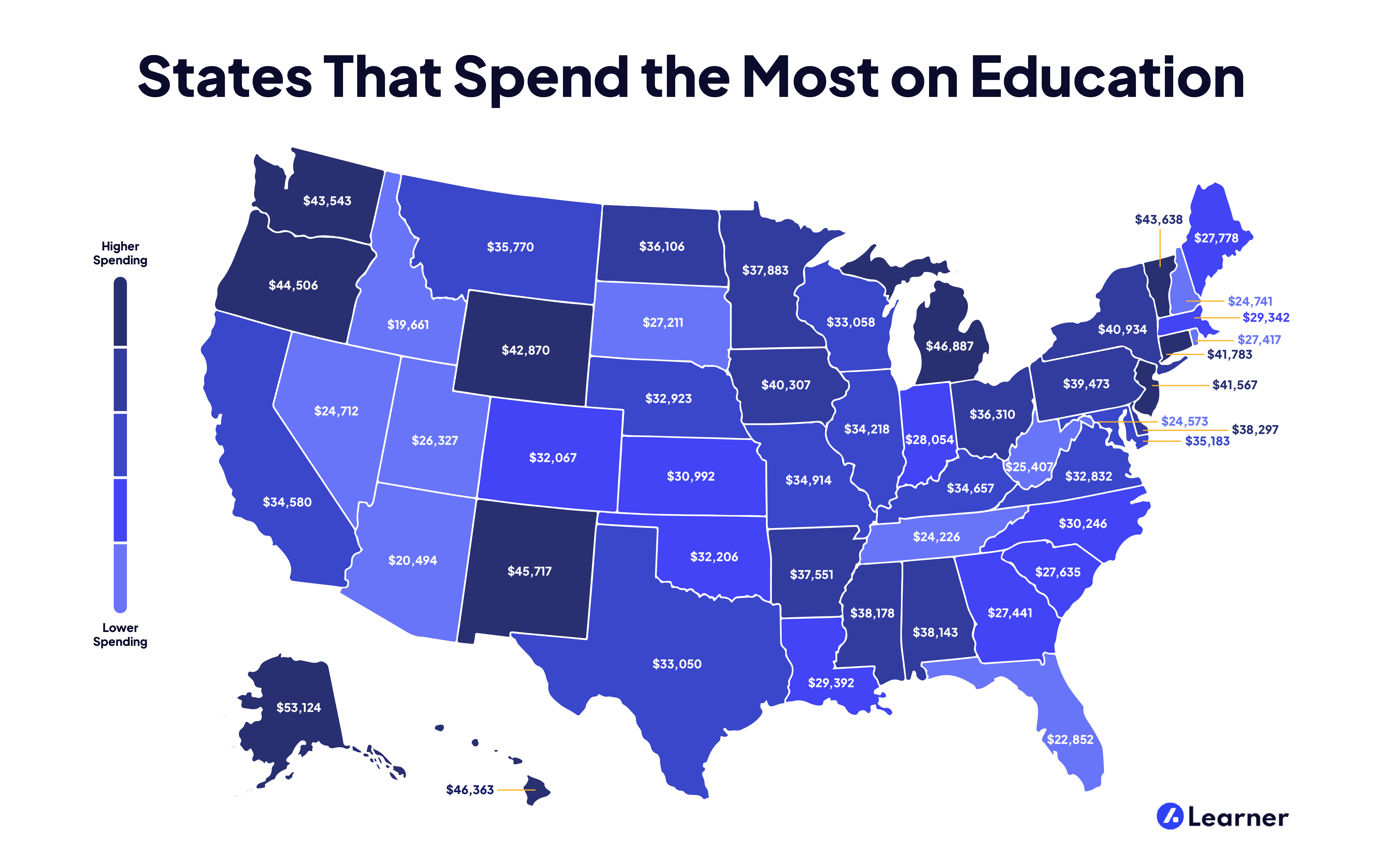### States That Spend the Most on Education

Investing in the future of our children via their education can help them build the lives they want. But education comes at a price—and a high one, at that. And some states invest more in their students than others. Find out which states have the highest spending per student!

Mathematics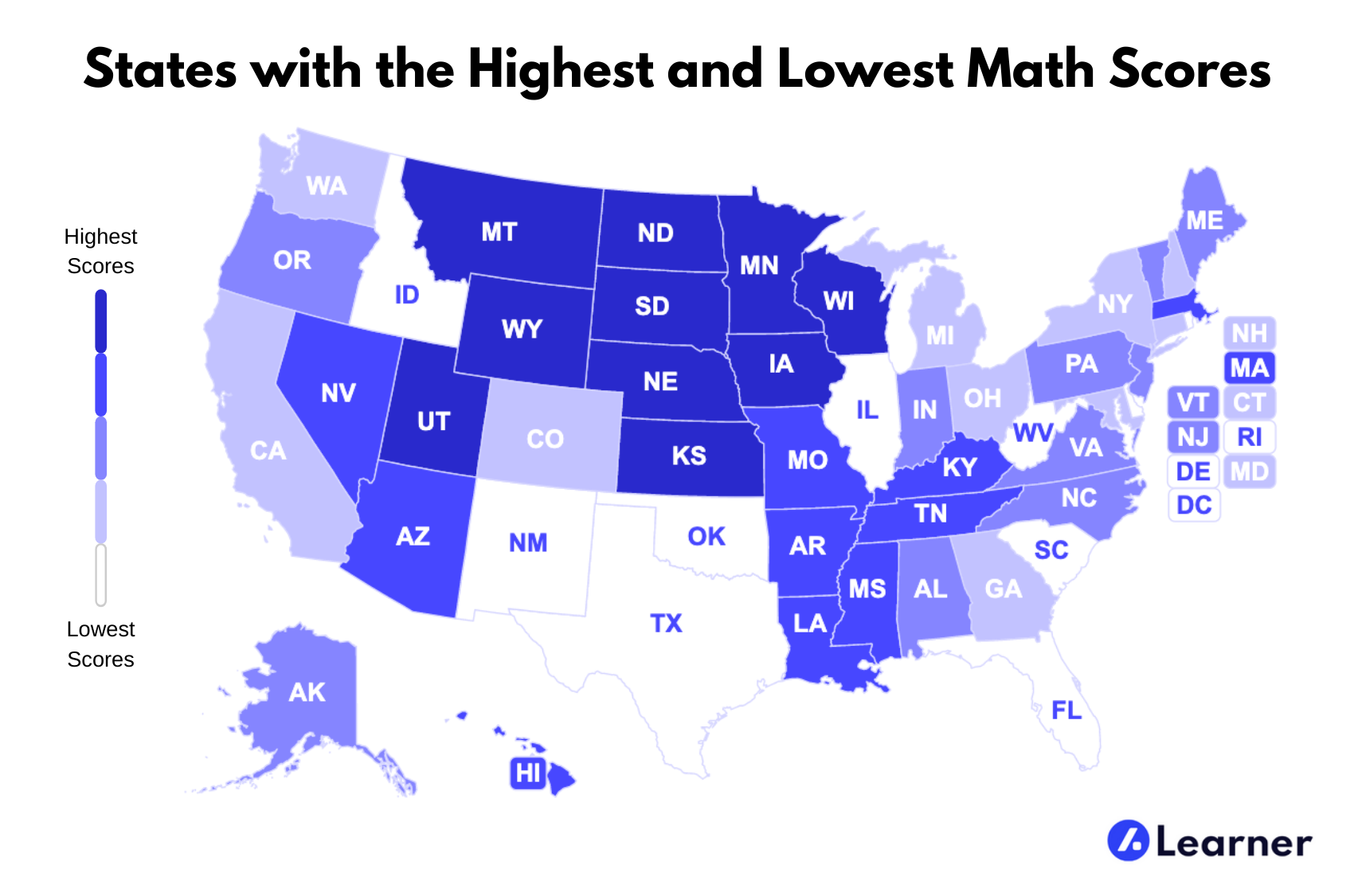### States with the Highest and Lowest Math Scores

At Learner, we understand both the difficulty and the necessity of math as a subject and wanted to know what math looks like in the United States. We took a look at the average SAT math scores throughout the nation to find which states had the highest and the lowest. Find out how your state stacks up against the rest!

Mathematics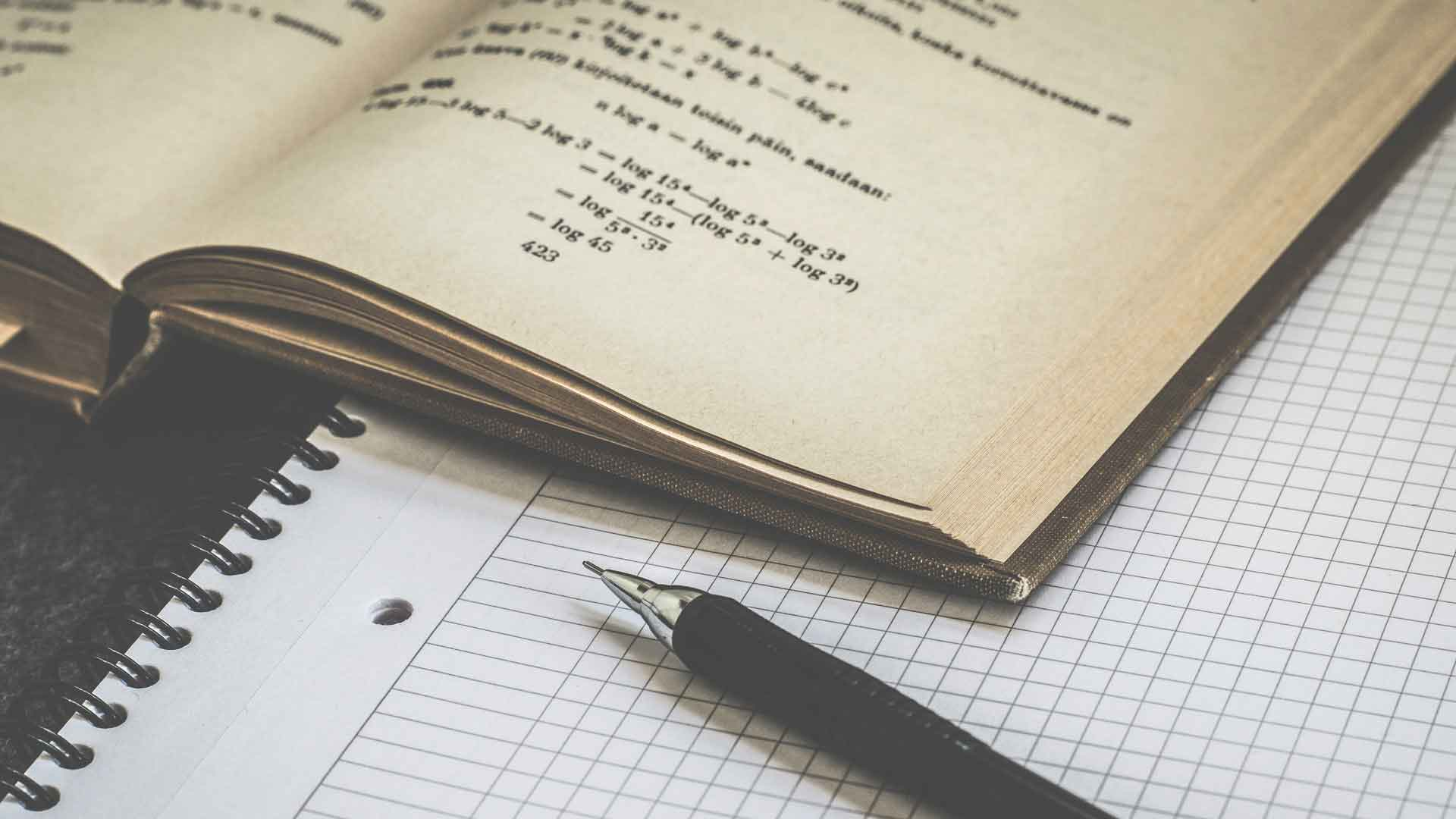### How Can I Score Well on the GED® Math Test?

Passing the General Education Development® (GED®) test gives a person the equivalent of a high school diploma. The math test is one of five sections of the exam. To score well on the GED® math test, you need a sound understanding of the concepts tested in each of the four content areas covered in this section.

Mathematics